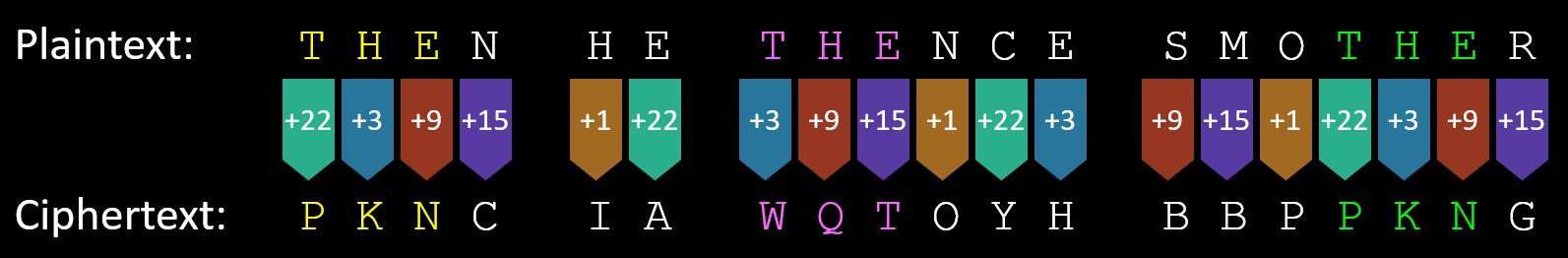# Vigenère Cipher – Finding the Key Length

No progress can be made in cracking a Vigenère cipher until the length of the key has been determined. We will look at two different ways of doing this.

#### Finding the key length by looking for repeating sequences of text

Below is a paragraph of text, taken from the previous page, and it has been encrypted using a Vigenère cipher with a key length of 13 characters.

XKIIMFUZZWPAJGWIUMBVNBEAJRRWIAXZKYBCRXMIGALXVCIXTHJAGLTKIFVNXIPYYPLIVEGJOCIDOBSWLHPPGILBTBJRKXKIGGILCSLQEUEFXSTNXHPBQMIXRJORVIXTMJRGFHGCOKPPCXJRGWRSBQTZTLNAIDGKXVGKKSDOCLHOHCKGYFBEUHKRFDGYVUQWTBCEUXRJWVGDPXWBSWLHWWZZERWJAEFXHVONYLWPBJWKMIXCHGMEARNHWSLXWPZEXHLJWHXKIZGTDIWXOXKINIMKYPDSROJHVHRHEGBHPALMSLHVGCXBTBYUSBIGFIVZETZNHPHRJXVEGKQTJWCV MCIOPJQWXBRWZLDXACQBHIQNZLKHRFGIFEWNAWRMQXSTKPIXWPMICRYOTKQGNRWKWSFVOEQFINXDVIMUWHRXLQANVMVXUCWPMQDLXAORYWLCYRLCVCQINIBMGDAQLTFRPOHHEZYOQWIQJXOEWIFHUOIWNVSPIQXBQZFRTQXALR WLSGDXBEUNEESYIHJKOTPANLVMQXVGIFEWNAXHBWXVGLFGHCQVHTUIGGTQHARWXKISPOKTQTMCCLHWHGCPJXCSOXYUXKVSRXBHTWCWDRGXVGZEXGMAISVHWSPZPTXOCLLWZIFGGJDCXJPSLDFSVOZRXYQIUAHACWRACDCRGHXK EHJGMETJAWPSVXCHZBCXWCLHGLTVGXQTMCJRGXKYGGDMTRCRXWSUIDTKPTCCNMQXKIDNGFCINGXEYWWIENXCPBBYPTWMCPCLJAMXROCEIFKMEIXWXRHSIXVGLLJGLJWHWKIFGGKSTENRWLDXWURXGVNUCECFSWPIFSTWLI

The encrypted text contains 846 characters, and, as stated, the key length was 13.

Normally, when applying a Vigenère cipher, the same word in English comes out differently in the ciphertext, and this is because it depends on which parts of the key are being used to encrypt it. In the example below, using the same Vigenère cipher from the previous page with a key length of 5, the three letters of 'THE' appear in the contrived phrase in the plaintext three times.In the first two occasions, the ciphertext comes out differently because each 'THE' is encrypted by a different part of the key. But, coincidentally, when it comes to the third occurrence of 'THE', it happens to fall at the same point in the key as the first occurrence and so the ciphertext is the same in both instances, i.e. 'PKN'.

When looking for the key length, this is what you hope for. You hope that the ciphertext is long enough so that, by chance, the same piece of English text gets encrypted by the same section of the key, thus generating the same ciphertext.

My example above has very obviously contrived English text, and you might wonder how likely it is that you will get the same section of plaintext encoded by the same section of the key. And the answer is that it happens surprisingly often. In fact, in the example in the box at the top of the screen, which was not contrived in any way (it was simply copied from the previous page), and which was encrypted with a long key of 13 characters (thus making it even less likely that we would get the repeating runs of text in the ciphertext that we are after), there is a section as long as seven characters that repeats itself:

XKIIMFUZZWPAJGWIUMBVNBEAJRRWIAXZKYBCRXMIGALXVCIXTHJAGLTKIFVNXIPYYPLIVEGJOCIDOBSWLHPPGILBTBJRKXKIGGILCSLQEUEFXSTNXHPBQMIXRJORVIXTMJRGFHGCOKPPCXJRGWRSBQTZTLNAIDGKXVGKKSDOCLHOHCKGYFBEUHKRFDGYVUQWTBCEUXRJWVGDPXWBSWLHWWZZERWJAEFXHVONYLWPBJWKMIXCHGMEARNHWSLXWPZEXHLJWHXKIZGTDIWXOXKINIMKYPDSROJHVHRHEGBHPALMSLHVGCXBTBYUSBIGFIVZETZNHPHRJXVEGKQTJWCV MCIOPJQWXBRWZLDXACQBHIQNZLKHRFGIFEWNAWRMQXSTKPIXWPMICRYOTKQGNRWKWSFVOEQFINXDVIMUWHRXLQANVMVXUCWPMQDLXAORYWLCYRLCVCQINIBMGDAQLTFRPOHHEZYOQWIQJXOEWIFHUOIWNVSPIQXBQZFRTQXALR WLSGDXBEUNEESYIHJKOTPANLVMQXVGIFEWNAXHBWXVGLFGHCQVHTUIGGTQHARWXKISPOKTQTMCCLHWHGCPJXCSOXYUXKVSRXBHTWCWDRGXVGZEXGMAISVHWSPZPTXOCLLWZIFGGJDCXJPSLDFSVOZRXYQIUAHACWRACDCRGHXK EHJGMETJAWPSVXCHZBCXWCLHGLTVGXQTMCJRGXKYGGDMTRCRXWSUIDTKPTCCNMQXKIDNGFCINGXEYWWIENXCPBBYPTWMCPCLJAMXROCEIFKMEIXWXRHSIXVGLLJGLJWHWKIFGGKSTENRWLDXWURXGVNUCECFSWPIFSTWLI

For reference, in this ciphertext, the phrase 'BSWLH' also occurs twice, and so does 'XVGL' and 'IFGG'. It is possible that these identical sequences have appeared completely by coincidence, i.e. they initially represented different sections of text in the plaintext and, when encrypted by different parts of the key, they just happened to come out as identical chunks of ciphertext. This is possible but it is unlikely, and it becomes very unlikely when you find repeating runs of text of 4 or more letters. When you have one as long as seven characters, then the most likely scenario is that these represent the same plaintext and they were concidentally encrypted with the same section of the key - as with the first and third 'THE's in the example above.

Given that this is the case, we can use what we have found to work out out the key length.

To find the repeating runs of text manually can be hard, but this website does it automatically for you. Click here and copy the ciphertext in the top box. Then, from the options just below it, select 'Perform Frequency Analysis' and click on the button. Scroll through the output to find the list of the longest repeating runs of text near the bottom, and, most helpfully of all, the gaps between them are given as well. See below for why this is important.

#### Using the discovered repeating sequences of text

If we count the number of characters between the repeating runs of text, this is what we find:

• GIFEWMA - number of characters between the two instances: 169
• BSWLH - number of characters between the two instances: 130
• XVGL - number of characters between the two instances: 247
• IFGG - number of characters between the two instances: 169
If two sections of the plaintext have been encrypted using the same section of the key, then this must mean that the gap between them must be an exact multiple of the key length. In my example with the three 'THE's above, notice how the two that have been encrypted into the same ciphertext (the first and third instances), have a gap of exactly 15 characters between them (do not count the spaces). Now look again at the bullet-points: the gaps between these repeated sections of texts are 130, 169 (twice) and 247. What is the only number apart from 1 that goes into all of them? The answer, of course, is 13. We can, therefore, be fairly confident that the key length behind this message is 13.

This is a nice, clear example. Sometimes, the results can be a little more ambiguous. Suppose the gaps had been as follows:

• GIFEWMA - number of characters between the two instances: 240
• BSWLH - number of characters between the two instances: 60
• XVGL - number of characters between the two instances: 180
• IFGG - number of characters between the two instances: 114

Whereas before, 13 had been the only common factor, there are three here (apart from 1): 2, 3, 6. When this happens, your first instinct should be to try the longest one first, i.e. 6, whilst remaining open to the possibility that it could be one of the shorter ones.

#### Next steps ...

Now that we have worked out the key length, we have made good progress, but before we look at the next step in fully cracking the Vigenère cipher, we will examine another way of working out the key length.

Cipher Challenge competition    Leave feedback